Courses
Courses for Kids
Free study material
Free LIVE classes
More

# JEE Advanced 2023 Revision Notes for Amino Acids and PeptidesLIVE
Join Vedantu’s FREE Mastercalss

Amino acids are the units that constitute proteins. They form bigger molecules by means of peptide linkage and make a protein molecule. These units are of different types and there are different features to distinguish them. This chapter introduces the definition of amino acids and their classifications. It also explains how amino acids form a protein with a proper illustration. To understand the concepts of this biomolecule, download and refer to Amino Acids and Peptides JEE Advanced notes for free from Vedantu.

These revision notes have been compiled with the sole aim to deliver a simpler version of all the fundamental principles of amino acids. These revision notes will help students learn the properties of an amino acid molecule and find out how thousands of these molecules form a protein. Refer to these notes to prepare well for the JEE Advanced exam.

Last updated date: 20th Sep 2023
Total views: 138.9k
Views today: 4.38k

## JEE Advanced 2023 Revision Notes Chemistry Amino Acids and Peptides

Amino acids are organic compounds containing both an amino group (-NH2) and carboxylic acid (-COOH) i.e., they are bi-functional

### Classification of Amino Acids:

Depending on the location of the amino group on the carbon chain that contains the carboxylic acid functional group, amino acids are classified as $\alpha ,\beta ,\gamma$ and $\delta$ etc.

• Though there are more than 700 different amino acids that occur naturally, only 20 of them are important

• When proteins are hydrolyzed only $\alpha -$ amino acids are obtained.

• The general formula of $\alpha -$ amino acids is $R - CH - COOH - N{H_2}$

Examples of $\alpha -$ amino acids are:

Glycine and Alanine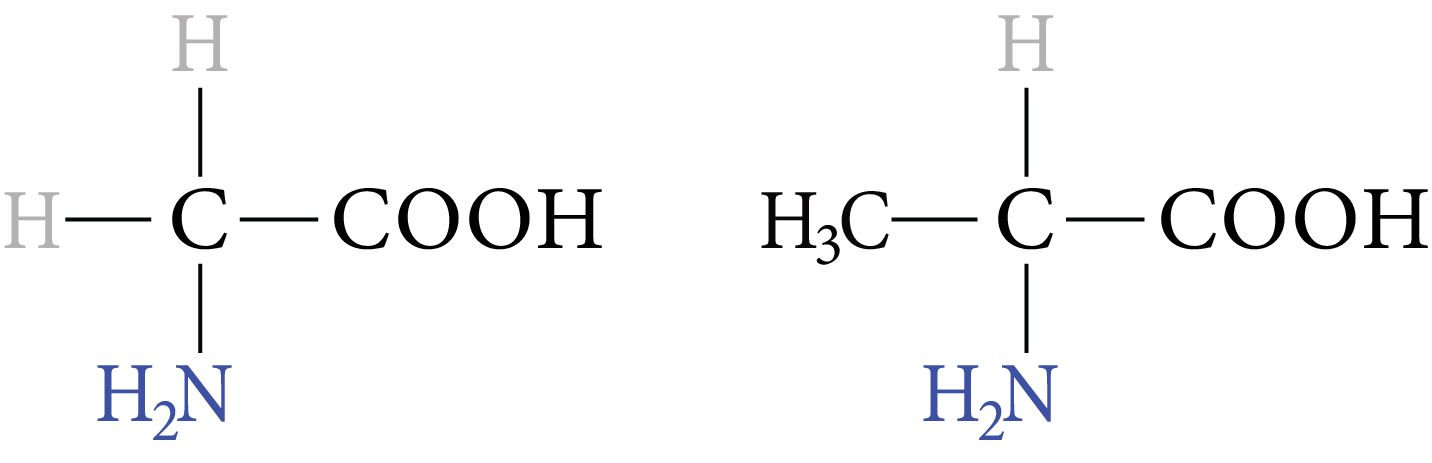Image: Glycine, Alanine

Example of $\beta -$ amino acids are:

${H_2}N - C{H_2} - C{H_2} - COOH$

$\beta -$ amino propionic acid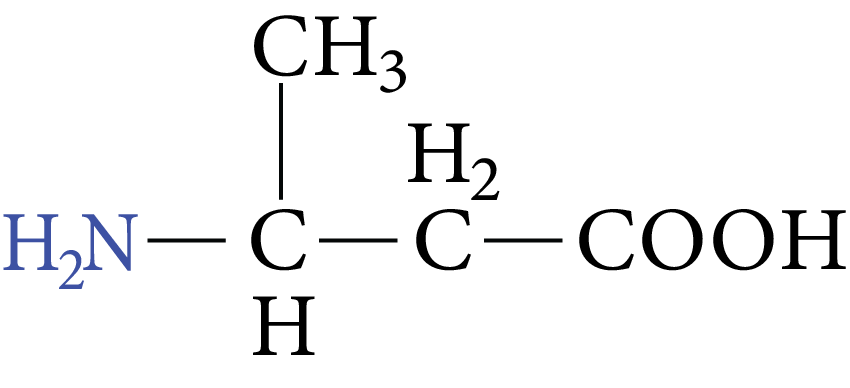Image: Amino Propionic acid

$\beta -$ amino butyric acidImage: Classification of amino acids: Amino Butyric Acid

$\gamma -$ amino pentanoic acid

Out of numerous amino acids, $\alpha -$ amino acids contain a primary amino group except for proline which contains a secondary amino groupImage: Classification of amino acid

Based on the relative number of $N{H_2}$ and $COOH$ groups.

The amino acids contain an equal number of $- N{H_2}$ and $- COOH$ are neutral amino acids. Ex: Glycine

If amino groups are more basic, Ex: Lysine, Arginine. If carboxylic groups are more acidic.

Ex: Aspartic acid, Glutamic acid.

### Based on the Source

• The amino acid, which cannot be synthesized in the body but can only be supplied to the body through diet, are called “essential amino acids”. They are valine, Leucine, Isoleucine, Arginine, Lysine, Threonine, Methionine, Phenylalanine, Tryptophan, and Histidine.

• The amino acid, which is synthesized in the body are known as a non-essential amino acid.

• Nearly all naturally occurring amino acids are amino acids containing amino groups on the carbon bonded to the carboxylic group.

Nomenclature: Amino acids are known by their common names and abbreviated by the first three letters or one letter symbol of their common names.

### Amino Acids With Non-Polar Side Are

 Amino Acid Characteristic of side-chain General symbol One letter code 1. Glycine $H$ Gly G 2. Alanine $- C{H_3}$ Ala A 3.Valine $- CH{\left( {C{H_3}} \right)_2}$ Val V 4.Leucine $- C{H_2} - CH{\left( {C{H_3}} \right)_2}$ Leu L 5.Iso Leucine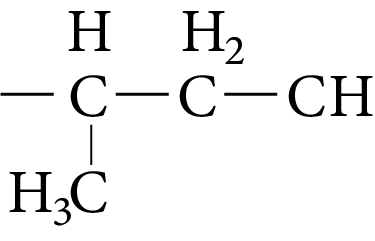Image: Iso leucine Ile I 6. Phenylalaninene $- C{H_2} - {C_6}{H_5}$ Phe F 7. Proline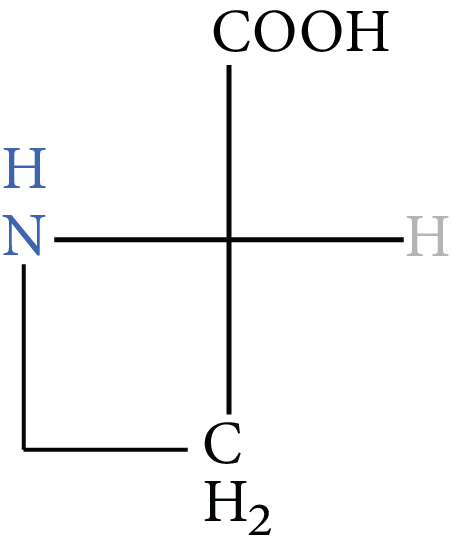Image: Proline Pro P

### Amino Acids With Polar But Neutral Side Chains Are

 Amino Acids Characteristic of Side-chain General Symbol One Letter Code 1. Tryptophan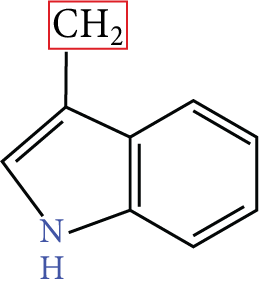Image: Amino acids Try w 2. Serine $- C{H_2}OH$ Ser S 3. Threonine $- C{H_2}OH - C{H_3}$ Thr T 4. Glutamine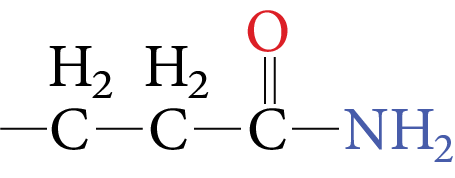Image: Glutamine Gin Q 5. Aspargine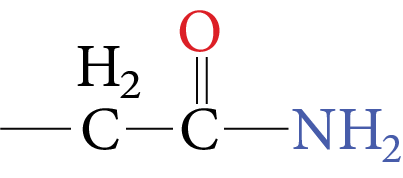Image: Aspargine Asn N 6.Cysteine $C{H_2}SH$ Cys C 7. Methionine $- C{H_2} - C{H_2} - SC{H_3}$ Met M 8. Tyrosine $- C{H_2} - {C_6}{H_5} - OH(para)$ Tyr Y

### Amino acid with Basic Side Chain Are:

 Amino Acids Characteristics of Side-chain General Symbol One Letter Vode Histidine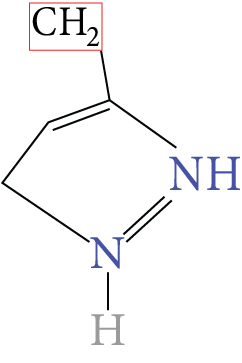Image: Histidine His H Arginine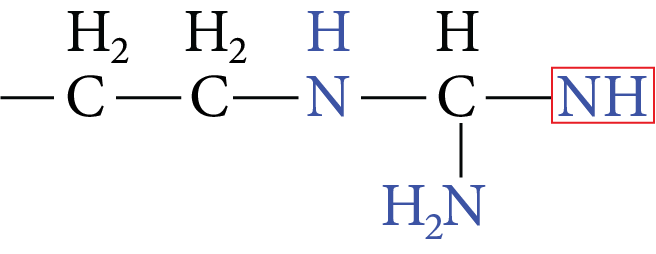Image: Arginine Arg R Lysine $- {(C{H_2})_4} - N{H_2}$ Lys K

### Amino Acid with Acidic Side Chain is:

 Amino acid Characteristics of side-chain General Symbol One letter code Glautamic acid $- C{H_2} - C{H_2} - COOH$ Glu E Aspartic acid $- C{H_2} - COOH$ Asp D

### Physical Properties of α-amino Acids

• The simplest amino acid is glycine, its IUPAC name is “2-amino ethanoic acid”

• Ther are generally colourless crystalline solids

• These are water-soluble, high melting solids and behave like salts rather than carboxylic acids or simple amines

• Except glycine, all other naturally occurring α-amino acid is optically active due to asymmetry at α-carbon

• Most of the naturally occurring amino acids with L-configuration

• They are highly polar and in aqueous solution, they form zwitter ions.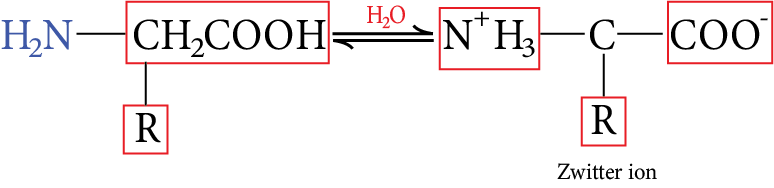Image: Zwitter ion

• In acidic solution, they form +ve ion, and in basic solution they form -ve ion.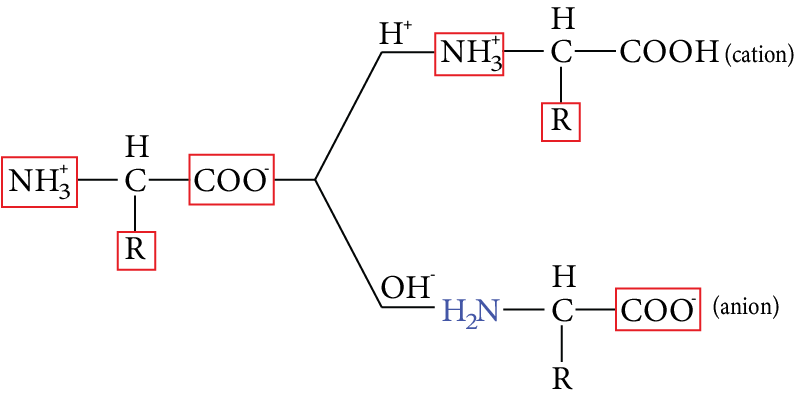Image: Zwitter ion contains two functional groups

• At a particular ${P^H}$, the dipolar ion acts as a neutral ion(isoelectric point)

• At a particular ${P^H}$, the dipolar ion of an amino acid (zwitterion) acts as a neutral ion and does not migrate to the cathode ‘or’ anode in an electric field, This ${P^H}$is known as isoelectric point of the amino acid

• The isoelectric point of neutral amino acid is calculated by ${P_1} = \dfrac{{P{K_{a1}} + P{K_{a2}}}}{2}$

• All amino acid does not have a same isoelectric point. An amino acid having more $COOH$groups will have ${P_1} < 7$

• An amino acid having more $N{H_2}$groups will have

Example: alanine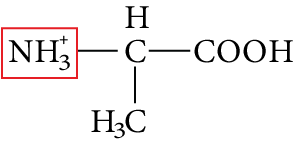Image: Alanine

${\text{COOH, }}{{\text{P}}^{{{\text{K}}_{\text{a}}}}}{\text{ = 2}}{\text{.34}}$

${\text{NH}}_{\text{3}}^{\text{ + }}{\text{, }}{{\text{P}}^{{{\text{K}}_{\text{a}}}}}{\text{ = 9}}{\text{.69}}$

Then, ${P_1} = \dfrac{{2.34 + 9.69}}{2} = 6.02$

• The isoelectric point depends on different groups present in the molecule of the amino acid.

• In neutral amino acids the ${P^H}$ range is 5.5 to 6.3

• At an isoelectric point, amino acids have the least solubility. So, it is used in the separation of different amino acids obtained from the hydrolysis of proteins.

### Peptide Bond:

The amide bond formed between the amino group of one amino acid and the carboxylic group of another amino acid by the loss of water is called a peptide bond.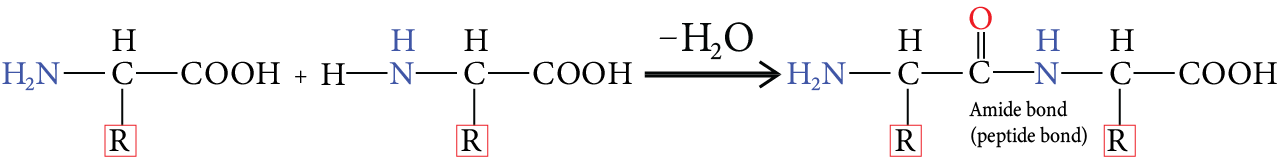Image: Peptide Bond

• The product obtained from two amino acid molecules through a peptide bond is called a dipeptide.

• Based on a number of amino acid molecules in peptides they are called tri, tetra, and polypeptides.

• Number of peptide linkages =((number of amino acid residues)-1)

• Shorter peptides(2-20amino acid) are called oligopeptides, longer peptides(more than 20 amino acids) are polypeptides.

• Proteins are polypeptides containing many amino acids whose molecular mass is more than 10,000. (Polypeptides contain more than 100 amino acids)

• Polypeptides are amphoteric

Ex: Silk, hair, skin, enzymes, hormones, etc

• Ina polypeptide structure free amino group $\left( {N{H_2}} \right)$ i.e., N-terminal residue is written on the left-hand side and the free carboxyl group on the right-hand side and the free carboxyl group on the right-hand side of the chain.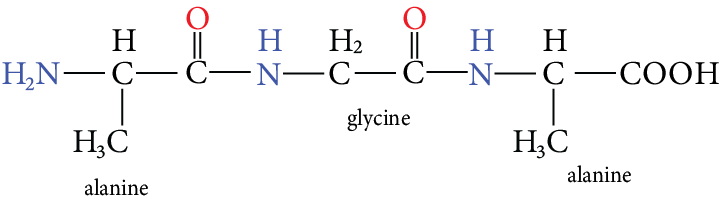Image: Alanyl glycyl alanine

The above structure has the name alanyl glycyl alanine.

• In the above structure the -COOH group is C-terminal residue and $- N{H_2}$ group is N-terminal residue

Most of the toxins in animal and plant venoms are proteins. A dipeptide called aspartame being 100 times sweeter than sucrose is used as a substitute for sugar.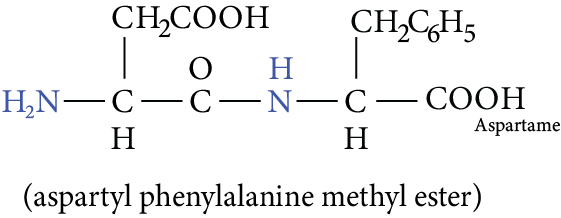Image: Aspartame

• The number of peptides possible for using different amino acids$= {n^m}$

• Here n is the number of amino acids, m is 2 for dipeptide, 3 for tripeptide, 4 for tetra peptide, etc.

A peptide is an amide formed by the intermolecular reaction of the amino group of one amino acid and the carboxyl group of the second amino acid. Dipeptides are made from two amino acids, tripeptides from three amino acids, etc, which may be the same or different. If there are four to ten amino acid residues, the peptide is called an oligopeptide. A polypeptide is a chain made up of many amino acids.

• By convention, the amino acid with the free amino group (N-terminus) is written at the left end and the one with the unreacted carboxyl group (C-terminus) at the right end. The suffix -in is replaced by -yl for each amino acid in the chain reading from left to right, followed by the full name of the C terminal amino acid.

• Sanger reagent, 1-fluoro-2, 4-dinitrobenzene (DNFB), is a reagent that reacts with a peptide chain Pep-$NHCOCHRN{H_3}^ +$. This reagent is used to identify the N-terminal AA of The peptide chain.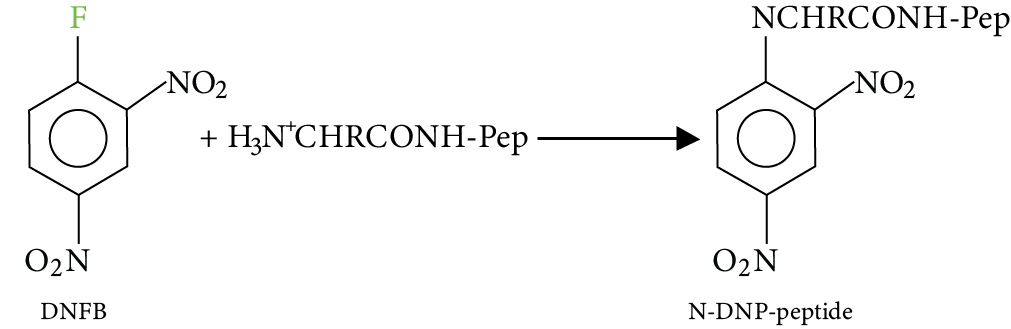Image: Dipeptides

Acid hydrolysis of the N-DNP-Peptide (where DNP is dinitrophenyl) gives individual amino acids and an N-2, 4- dinitrophenyl amine acid (N-DNP-AA, where AA is N-terminal), detected by its yellow colour.

## Importance of Amino Acids and Peptides

Amino acids, as mentioned earlier, are a class of bio-molecules that constitute different types of proteins. These amino acids can be found in living organisms. They are the prime constituents of life, forming different cell organelles, tissues, organs, and organ systems at the micro-level.

This chapter will teach students the different structures of amino acids, where they can learn the configuration of amino acids and how they form different types of protein. There are two types of classification according to the functions and compositions of this bio-molecule.

This chapter is quite important for the preparation for JEE Advanced exam. Students will need in-depth knowledge to answer fundamental questions. These revision notes ensure that you get the best understanding of the concepts of amino acids and peptides.

It also introduces the classification of proteins according to their functions and structures. The types of proteins are explained using images and proper illustrations with examples. Hence, this chapter is quite important for scoring good marks in the IIT JEE exams.

## Benefits of Amino Acids and Peptides JEE Advanced Notes PDF

• These notes have been prepared by the top experts at Vedantu to explain all the concepts related to amino acids, peptide bonds, and protein molecules. The simple explanation will give you the best medium to study this chapter and understand the fundamental concepts easily.

• Amino Acids and Peptides JEE Advanced revision notes will become much easier when you get concise revision notes for every section of this chapter. It will thus reduce the time to studying and revising the chapter using these notes. Also, you will be able to comprehend the concepts and focus on clarifying your doubts with the help of these notes.

• Recall what you have studied while revising before the exam using these notes. The concise and easy format of these revision notes will help you remember what you have studied effectively. Hence, you can easily make your preparation better without investing more time.

• Solve the sample questions given in the revision notes to assess your preparation. Evaluate your answering skills for this chapter and become better by focusing on the weak sections of this chapter.

Students strive to find the best revision notes to add to their study material and make chemistry preparation better. Download the Amino Acids and Peptides JEE Advanced revision PDF for free and prepare this chapter for the exam. Make your study sessions more fruitful by referring to these notes and revising them efficiently.

## FAQs on JEE Advanced 2023 Revision Notes for Amino Acids and Peptides

1. What are amino acids?

They are organic compounds that are chemically defined as the derivatives of carboxylic acid. A molecule contains an amino and a carboxylic group. This is why it is named amino acid.

2. What are basic amino acids?

When an amino acid molecule contains a basic functional group apart from the amino and carboxylic groups, it is called a basic amino acid. Example: Histidine and Lycine.

3. What is an acidic amino acid?

An acidic amino acid contains an acidic group apart from the carboxyl group present in the molecule. Example: Glutamic Acid.

4. Are amino acids soluble in water?

Amino acids are highly soluble in water but not in organic solvents.